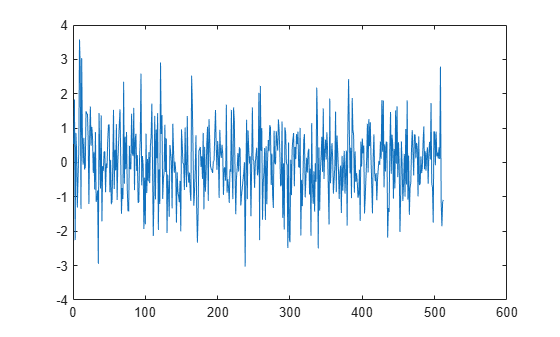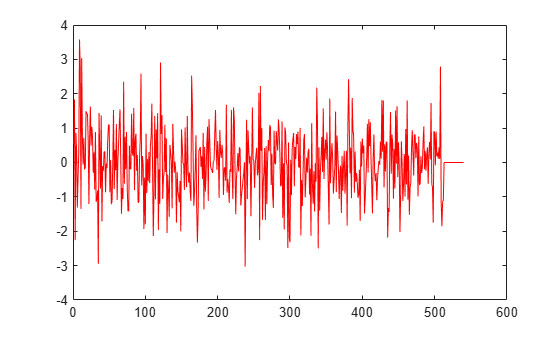# info

Get cumulative overrun and underrun

## Syntax

``S = info(asyncBuff)``

## Description

example

````S = info(asyncBuff)` returns a structure, `S`, containing the cumulative overrun and underrun information of the `dsp.AsyncBuffer` System object™, `asyncBuff`.```

## Examples

collapse all

The `dsp.AsyncBuffer` System object™ supports reading variable frame sizes from the buffer.

Create a `dsp.AsyncBuffer` System object. The input is white Gaussian noise with a mean of 0, a standard deviation of 1, and a frame size of 512 samples. Write the input to the buffer using the `write` method.

```asyncBuff = dsp.AsyncBuffer; input = randn(512,1); write(asyncBuff,input); plot(input) hold on```Store the data that is read from the buffer in `outTotal`.

Plot the input signal and data that is read from the buffer in the same plot. Read data from the buffer until all samples are read. In each iteration of the loop, `randi` determines the number of samples to read. Therefore, the signal is read in as a variable-size signal. The `prevIndex` variable keeps track of the previous index value that contains the data.

```outTotal = zeros(size(input)); prevIndex = 0; while asyncBuff.NumUnreadSamples ~= 0 numToRead = randi([1,64]); out = read(asyncBuff,numToRead); outTotal(prevIndex+1:prevIndex+numToRead) = out; prevIndex = prevIndex+numToRead; end plot(outTotal,'r') hold off```Verify that the input data and the data read from the buffer (excluding the underrun samples, if any) are the same. The cumulative number of overrun and underrun samples in the buffer is determined by the `info` function.

`S = info(asyncBuff)`
```S = struct with fields: CumulativeOverrun: 0 CumulativeUnderrun: 28 ```

The `CumulativeUnderrun` field shows the number of samples underrun per channel. Underrun occurs if you attempt to read more samples than available.

## Input Arguments

collapse all

Async buffer, specified as a `dsp.AsyncBuffer` System object.

## Output Arguments

collapse all

Cumulative overrun and underrun information, returned as a structure. The fields for `S` are described in the table.

FieldValue
`CumulativeOverrun`

Number of samples overrun per channel since last call to `reset`. The number of samples overrun is the number of unread samples overwritten.

`CumulativeUnderrun`

Number of samples underrun per channel since last call to `reset`. Underrun occurs if you attempt to read more samples than available.

The `CumulativeOverrun` and `CumulativeUnderrun` properties are data type `int32`.

## Version History

Introduced in R2017a